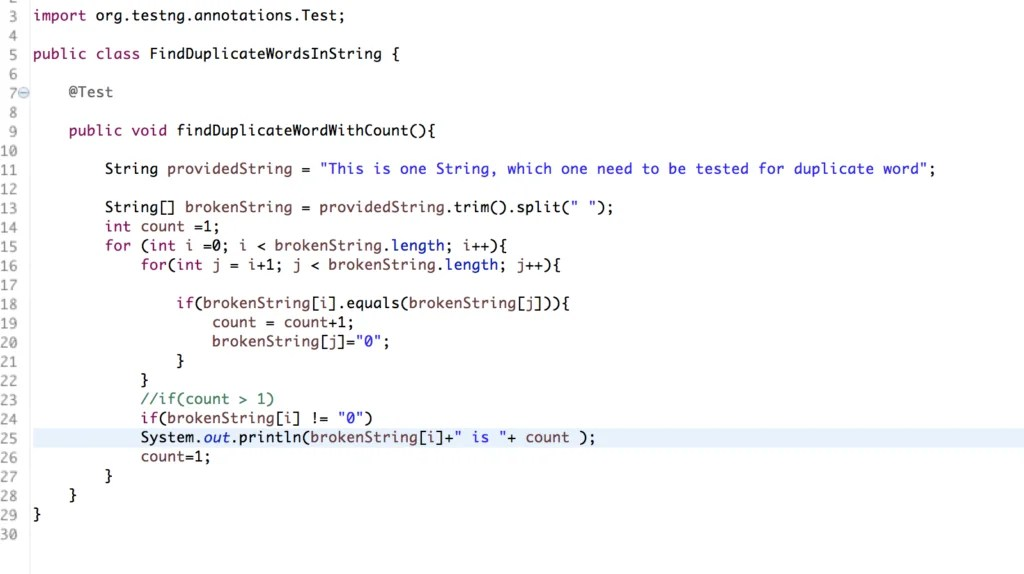Home » Java Tutorial » 5 Commonly Asked Java String Question in Selenium Interview

# 5 Commonly Asked Java String Question in Selenium Interview

Nowadays interview’s conducted for Automation Engineers are having lots of Java Question. Sometimes the same question with small changes is asked which have multiple approaches to implement. One of the most favourite parts in java for Automation Engineers is String.So here I am listing top 5 String questions asked in Interview and I am pretty sure you would find it helpful and will answer these question easily if asked by an interviewer.

But if you want to learn about String in Java, then I would suggest you, read Exploring String class in Java.

For more java tutorial I would suggest reading Java Tutorial.

Question 1: Write a program to reverse a string.

Input String:  “This is the string to reverse”

Final Output: “esrever ot gnirts eht si sihT”

The above question can be answered in many ways but here I am going to post two solutions. In which one is the simplest without giving much stress to mind just by using basic java concept and the second one is the most efficient that I have found(This solution can be used in reversing array as well)

Solution 1:

• First, we will receive the string (Either in form of variable or Scanner method)
• We will find the length of the string.
• We will use StringBuilder and will append the last character as the first character

Note:  In all my program I am using TestNG (@Test).

Here is the output on the console:

Solution 2:

1. First, We will take String(In this example we will take the string in one variable ) and will convert String into StringBuilder.
2. After conversion from String to StringBuffer, We will find the length of StringBuilder.
3. Finally, we will swap characters from both ends until we will reach to mid of string.

Here is the code :

Here is the output on the console:

Create a Function which takes String and gives output as reversed String.

Question 2: In the above question 1, we have been asked to reverse complete string character by character but at the same time interviewer might ask to reverse words in place of each character like this.

Input String: “This is the string to reverse”

Output String: “reverse to string the is This”

This question again going to have many approaches but I am going to post the most optimal way where we will use the split function of string.

Solution:

1. Take the String (In this example we are going to use a variable in place of Scanner class) and will break the string in an array using the split function of String using regular expression space “\\s”.
2. We will find the length of the array and will iterate the array in reverse.

Here is the output on the console:

Question 3: Find duplicate characters in the provided String and also find the count of each duplicate character.

Input String: “Bharat”

Output: a

If the above question was asked to find only the duplicate character then it was just a simple question that can be solved using the loop and some of the concept of an array. But as soon as the count is added with this question it becomes a very good question to solve.

Solution: For this first, we need to find duplicate value and its count where the character could be unique but its count might change with each iteration. So here we need to use hashmap where the key will remain constant and could not be duplicate but its value could be updated using its key.

1. We will take the String.
2. We will convert it in character array.
3. We will define one hashmap where each character would act as a key and its count as value. Here we will check the presence of the key in the map and if the key exists we will increase the count of character or if it doesn’t present as the key in the map we will add it.
4. In the end, we will print the key and value pair where the key would be duplicate character and value would be its count.

Note:  Here I am assuming that lower case and upper case are being counted as same

But if you want to count both separately then replace this line of code

to this

So here is the code

Here is the output on console(Pasting extract of the console)

Question 4:

In Java write one program which finds duplicate words in the string and also find the count of the word.

This question can be solved in the same ways as it was done for the duplicate character. The only thing that we need to incorporate is to split the string using space.

Solution:

1. We will take the provided string and will split it using space in the string array.
2. After this, we will compare each word from the place of word index+1 to the length-1.
3. If we would find any value at both indexes in the array then we will increase the count.
4. On the basis of the count, we will print the duplicate word.

Here is the code:

Output in the console.

But if the interviewer asks for the count of each word present in the sentence then in place of this line of code

with this line

then out of the above code would be like this

So we can see that the count of each word is given in output.

Question 5:

How you will find that these two Strings are equal.

String firstString =  “Hello”

String secondString = new String(“Hello”)